# fode1.ppt

Mar. 25, 2023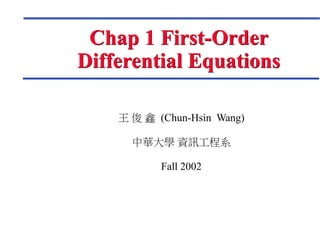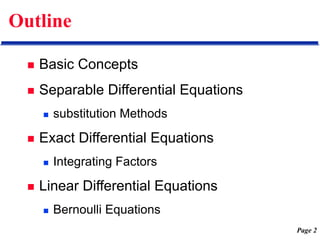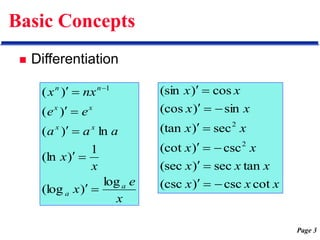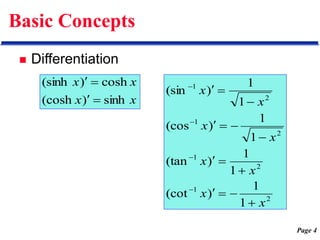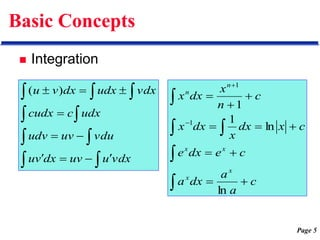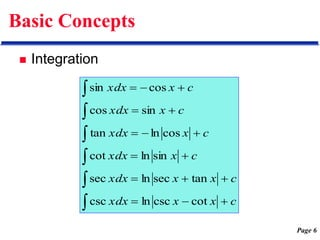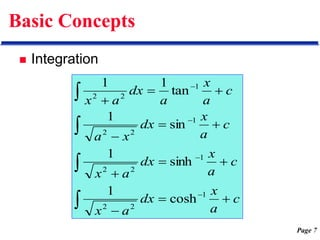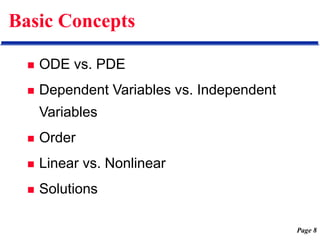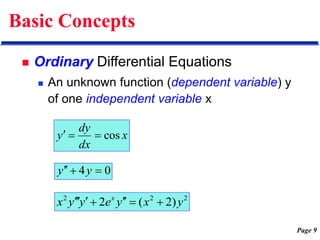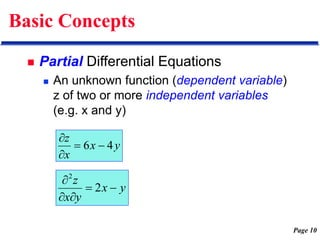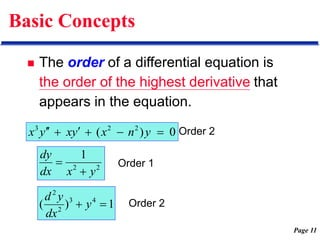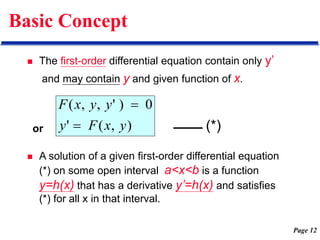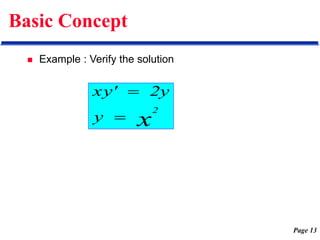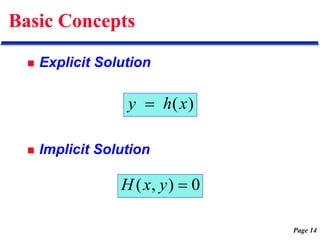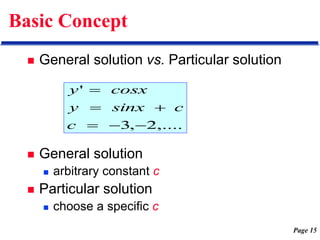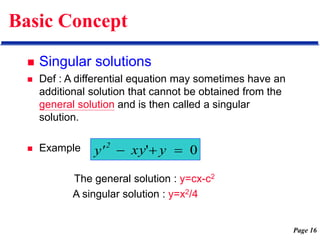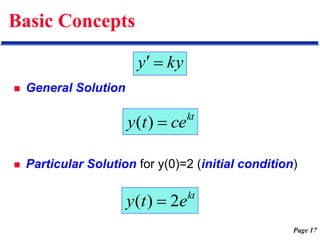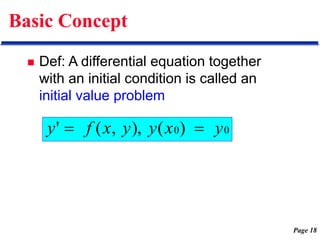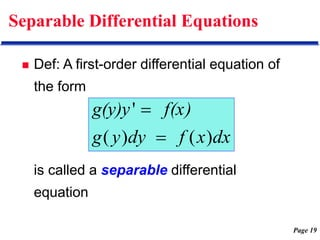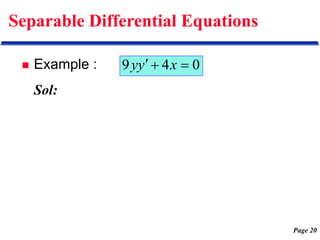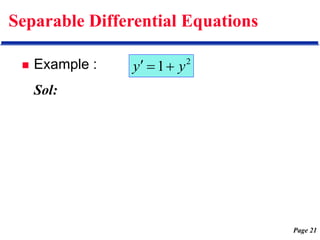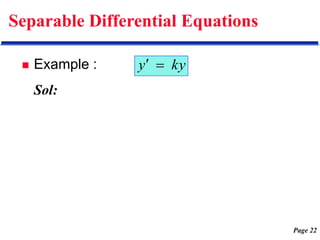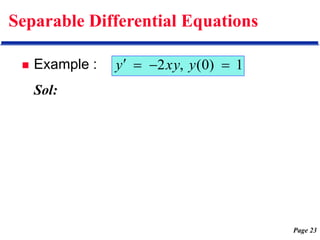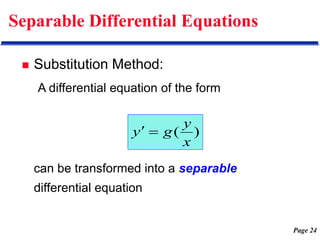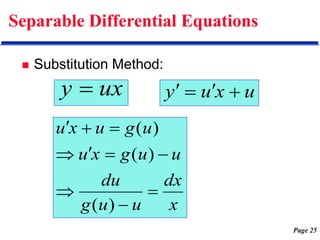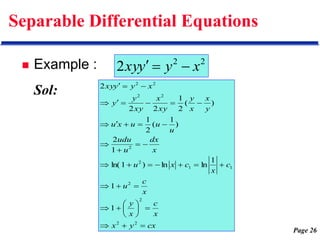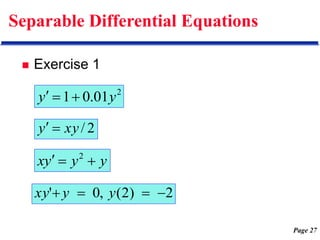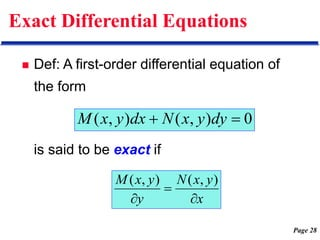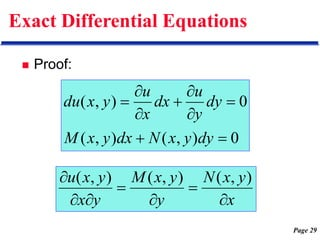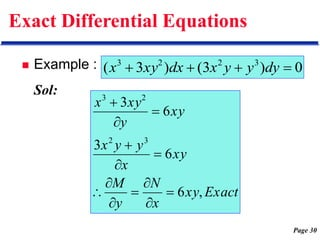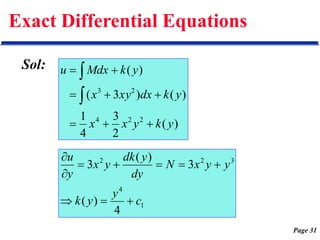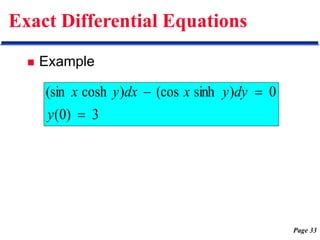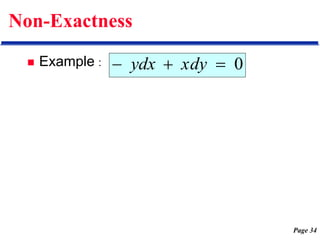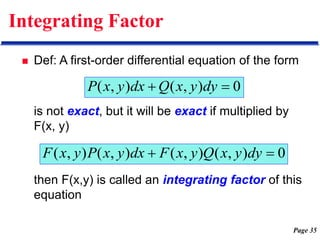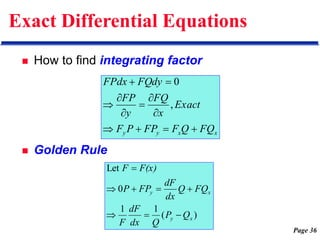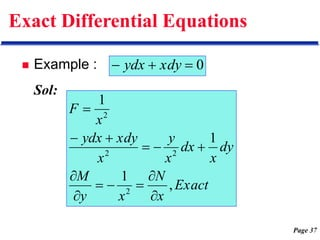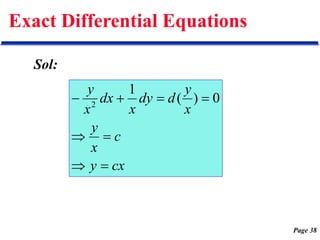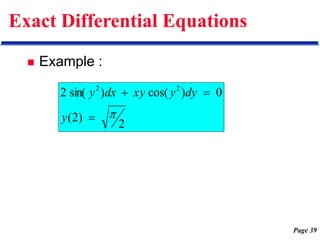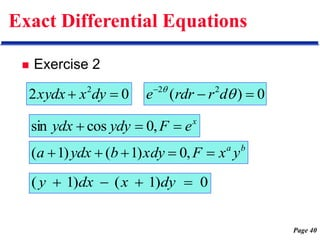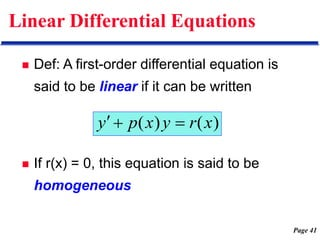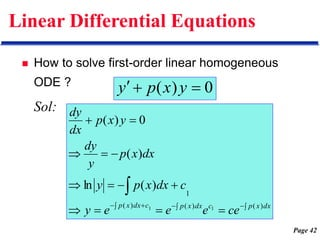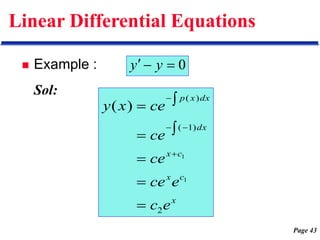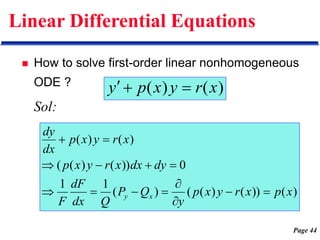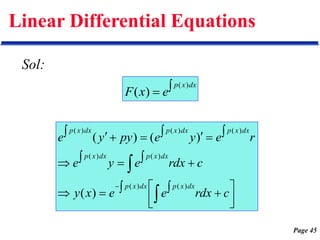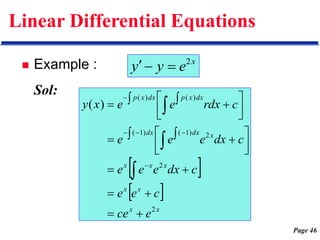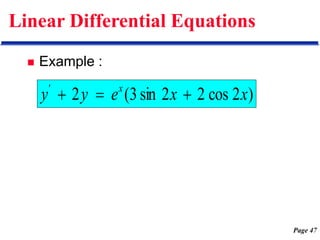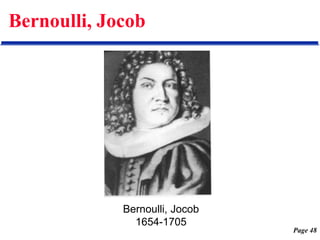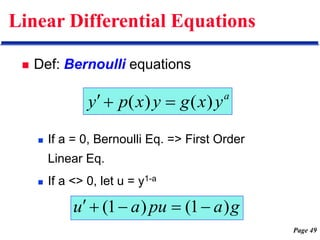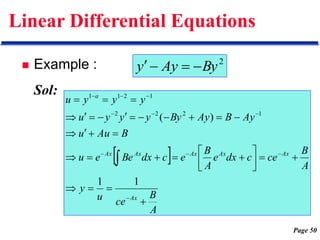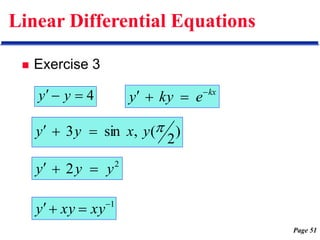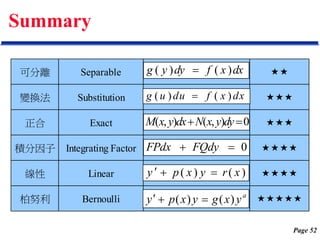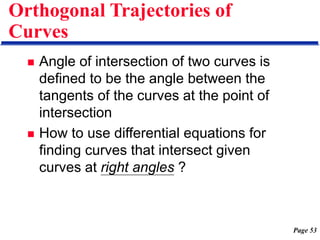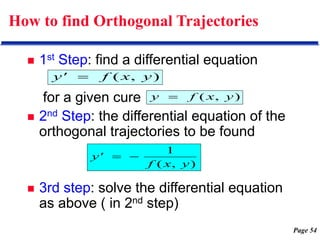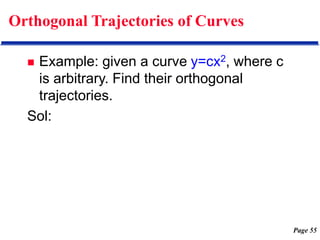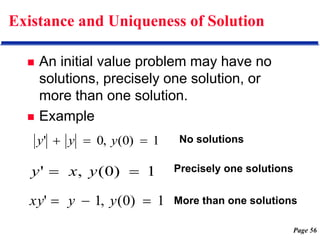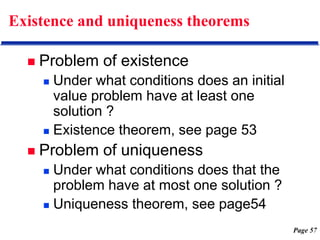1 of 57

### fode1.ppt

1. 王 俊 鑫 (Chun-Hsin Wang) 中華大學 資訊工程系 Fall 2002 Chap 1 First-Order Differential Equations
2. Page 2 Outline  Basic Concepts  Separable Differential Equations  substitution Methods  Exact Differential Equations  Integrating Factors  Linear Differential Equations  Bernoulli Equations
3. Page 3 Basic Concepts  Differentiation x e x x x a a a e e nx x a a x x x x n n log ) (log 1 ) (ln ln ) ( ) ( ) ( 1            x x x x x x x x x x x x x x cot csc ) (csc tan sec ) (sec csc ) (cot sec ) (tan sin ) (cos cos ) (sin 2 2               
4. Page 4 Basic Concepts  Differentiation x x x x sinh ) (cosh cosh ) (sinh     2 1 2 1 2 1 2 1 1 1 ) (cot 1 1 ) (tan 1 1 ) (cos 1 1 ) (sin x x x x x x x x                  
5. Page 5 Basic Concepts  Integration c a a dx a c e dx e c x dx x dx x c n x dx x x x x x n n                  ln ln 1 1 1 1                    vdx u uv dx v u vdu uv udv udx c cudx vdx udx dx v u ) (
6. Page 6 Basic Concepts  Integration c x x xdx c x x xdx c x xdx c x xdx c x xdx c x xdx                       cot csc ln csc tan sec ln sec sin ln cot cos ln tan sin cos cos sin
7. Page 7 Basic Concepts  Integration c a x dx a x c a x dx a x c a x dx x a c a x a dx a x                     1 2 2 1 2 2 1 2 2 1 2 2 cosh 1 sinh 1 sin 1 tan 1 1
8. Page 8 Basic Concepts  ODE vs. PDE  Dependent Variables vs. Independent Variables  Order  Linear vs. Nonlinear  Solutions
9. Page 9 Basic Concepts  Ordinary Differential Equations  An unknown function (dependent variable) y of one independent variable x x dx dy y cos    0 4     y y 2 2 2 ) 2 ( 2 y x y e y y x x         
10. Page 10 Basic Concepts  Partial Differential Equations  An unknown function (dependent variable) z of two or more independent variables (e.g. x and y) y x x z 4 6     y x y x z      2 2
11. Page 11 Basic Concepts  The order of a differential equation is the order of the highest derivative that appears in the equation. 0 ) ( 2 2 3        y n x y x y x Order 2 2 2 1 y x dx dy   Order 1 1 ) ( 4 3 2 2   y dx y d Order 2
12. Page 12 Basic Concept  The first-order differential equation contain only y’ and may contain y and given function of x.  A solution of a given first-order differential equation (*) on some open interval a<x<b is a function y=h(x) that has a derivative y’=h(x) and satisfies (*) for all x in that interval. ) , ( ' 0 ) ' , , ( y x F y y y x F   or (*)
13. Page 13 Basic Concept  Example : Verify the solution x 2 y 2y xy'  
14. Page 14 Basic Concepts  Explicit Solution  Implicit Solution ) (x h y  0 ) , (  y x H
15. Page 15 Basic Concept  General solution vs. Particular solution  General solution  arbitrary constant c  Particular solution  choose a specific c ,.... 2 , 3 '       c c sinx y cosx y
16. Page 16 Basic Concept  Singular solutions  Def : A differential equation may sometimes have an additional solution that cannot be obtained from the general solution and is then called a singular solution.  Example The general solution : y=cx-c2 A singular solution : y=x2/4 0 '    y xy y' 2
17. Page 17 Basic Concepts  General Solution  Particular Solution for y(0)=2 (initial condition) kt ce t y  ) ( kt e t y 2 ) (  ky y  
18. Page 18 Basic Concept  Def: A differential equation together with an initial condition is called an initial value problem 0 0) ( ), , ( ' y x y y x f y  
19. Page 19 Separable Differential Equations  Def: A first-order differential equation of the form is called a separable differential equation dx x f dy y g f(x) g(y)y ) ( ) ( '  
20. Page 20 Separable Differential Equations  Example : Sol: 0 4 9    x y y
21. Page 21 Separable Differential Equations  Example : Sol: 2 1 y y   
22. Page 22 Separable Differential Equations  Example : Sol: ky y  
23. Page 23 Separable Differential Equations  Example : Sol: 1 ) 0 ( , 2     y xy y
24. Page 24 Separable Differential Equations  Substitution Method: A differential equation of the form can be transformed into a separable differential equation ) ( x y g y  
25. Page 25 Separable Differential Equations  Substitution Method: ux y  u x u y     x dx u u g du u u g x u u g u x u           ) ( ) ( ) (
26. Page 26 Separable Differential Equations  Example : Sol: 2 2 2 x y y xy    cx y x x c x y x c u c x c x u x dx u udu u u u x u y x x y xy x xy y y x y y xy                                         2 2 2 2 1 1 2 2 2 2 2 2 1 1 1 ln ln ) 1 ln( 1 2 ) 1 ( 2 1 ) ( 2 1 2 2 2
27. Page 27 Separable Differential Equations  Exercise 1 2 01 . 0 1 y y    2 / xy y   y y y x    2 2 ) 2 ( , 0 '     y y xy
28. Page 28 Exact Differential Equations  Def: A first-order differential equation of the form is said to be exact if 0 ) , ( ) , (   dy y x N dx y x M x y x N y y x M    ) , ( ) , (
29. Page 29 Exact Differential Equations  Proof: 0 ) , ( ) , ( 0 ) , (          dy y x N dx y x M dy y u dx x u y x du x y x N y y x M y x y x u        ) , ( ) , ( ) , (
30. Page 30 Exact Differential Equations  Example : Sol: 0 ) 3 ( ) 3 ( 3 2 2 3     dy y y x dx xy x Exact xy x N y M xy x y y x xy y xy x , 6 6 3 6 3 3 2 2 3             
31. Page 31 Exact Differential Equations Sol: ) ( 2 3 4 1 ) ( ) 3 ( ) ( 2 2 4 2 3 y k y x x y k dx xy x y k Mdx u           1 4 3 2 2 4 ) ( 3 ) ( 3 c y y k y y x N dy y dk y x y u          
32. Page 32 Exact Differential Equations Sol: c y y x x y x u     ) 6 ( 4 1 ) , ( 4 2 2 4
33. Page 33 Exact Differential Equations  Example 3 ) 0 ( 0 ) sinh (cos ) cosh (sin    y dy y x dx y x
34. Page 34 Non-Exactness  Example : 0    xdy ydx
35. Page 35 Integrating Factor  Def: A first-order differential equation of the form is not exact, but it will be exact if multiplied by F(x, y) then F(x,y) is called an integrating factor of this equation 0 ) , ( ) , (   dy y x Q dx y x P 0 ) , ( ) , ( ) , ( ) , (   dy y x Q y x F dx y x P y x F
36. Page 36 Exact Differential Equations  How to find integrating factor  Golden Rule x x y y FQ Q F FP P F Exact x FQ y FP FQdy FPdx             , 0 ) ( 1 1 0 Let x y x y Q P Q dx dF F FQ Q dx dF FP P F(x) F        
37. Page 37 Exact Differential Equations  Example : Sol: 0    xdy ydx Exact x N x y M dy x dx x y x xdy ydx x F , 1 1 1 2 2 2 2             
38. Page 38 Exact Differential Equations Sol: cx y c x y x y d dy x dx x y         0 ) ( 1 2
39. Page 39 Exact Differential Equations  Example : 2 ) 2 ( 0 ) cos( ) sin( 2 2 2     y dy y xy dx y
40. Page 40 Exact Differential Equations  Exercise 2 0 2 2   dy x xydx 0 ) ( 2 2      d r rdr e x e F ydy ydx    , 0 cos sin b a y x F xdy b ydx a      , 0 ) 1 ( ) 1 ( 0 ) 1 ( ) 1 (     dy x dx y
41. Page 41 Linear Differential Equations  Def: A first-order differential equation is said to be linear if it can be written  If r(x) = 0, this equation is said to be homogeneous ) ( ) ( x r y x p y   
42. Page 42 Linear Differential Equations  How to solve first-order linear homogeneous ODE ? Sol: 0 ) (    y x p y                      dx x p c dx x p c dx x p ce e e e y c dx x p y dx x p y dy y x p dx dy ) ( ) ( ) ( 1 1 1 ) ( ln ) ( 0 ) (
43. Page 43 Linear Differential Equations  Example : Sol: 0    y y x c x c x dx dx x p e c e ce ce ce ce x y 2 ) 1 ( ) ( 1 1 ) (           
44. Page 44 Linear Differential Equations  How to solve first-order linear nonhomogeneous ODE ? Sol: ) ( ) ( x r y x p y    ) ( )) ( ) ( ( ) ( 1 1 0 )) ( ) ( ( ) ( ) ( x p x r y x p y Q P Q dx dF F dy dx x r y x p x r y x p dx dy x y              
45. Page 45 Linear Differential Equations Sol:   dx x p e x F ) ( ) (                            c dx r e e x y c dx r e y e r e y e py y e dx x p dx x p dx x p dx x p dx x p dx x p dx x p ) ( ) ( ) ( ) ( ) ( ) ( ) ( ) ( ) ( ) (
46. Page 46 Linear Differential Equations  Example : Sol: x e y y 2        x x x x x x x x dx dx dx x p dx x p e ce c e e c dx e e e c dx e e e c dx r e e x y 2 2 2 ) 1 ( ) 1 ( ) ( ) ( ) (                                  
47. Page 47 Linear Differential Equations  Example : ) 2 cos 2 2 sin 3 ( 2 x x e y y x '   
48. Page 48 Bernoulli, Jocob Bernoulli, Jocob 1654-1705
49. Page 49 Linear Differential Equations  Def: Bernoulli equations  If a = 0, Bernoulli Eq. => First Order Linear Eq.  If a <> 0, let u = y1-a a y x g y x p y ) ( ) (    g a pu a u ) 1 ( ) 1 (     
50. Page 50 Linear Differential Equations  Example : Sol: 2 By Ay y       A B ce u y A B ce c dx e A B e c dx Be e u B Au u Ay B Ay By y y y u y y y u Ax Ax Ax Ax Ax Ax a                                               1 1 ) ( 1 2 2 2 1 2 1 1
51. Page 51 Linear Differential Equations  Exercise 3 4    y y kx e ky y     2 2 y y y    1     xy xy y ) 2 ( , sin 3  y x y y   
52. Page 52 Summary 可分離 Separable  變換法 Substitution  正合 Exact  積分因子 Integrating Factor  線性 Linear  柏努利 Bernoulli  dx x f dy y g ) ( ) (  dx x f du u g ) ( ) (  0 ) , ( ) , (   dy y x N dx y x M 0   FQdy FPdx ) ( ) ( x r y x p y    a y x g y x p y ) ( ) (   
53. Page 53 Orthogonal Trajectories of Curves  Angle of intersection of two curves is defined to be the angle between the tangents of the curves at the point of intersection  How to use differential equations for finding curves that intersect given curves at right angles ?
54. Page 54 How to find Orthogonal Trajectories  1st Step: find a differential equation for a given cure  2nd Step: the differential equation of the orthogonal trajectories to be found  3rd step: solve the differential equation as above ( in 2nd step) ) , ( y x f y  ) , ( y x f y'  ) , ( 1 y x f y'  
55. Page 55 Orthogonal Trajectories of Curves  Example: given a curve y=cx2, where c is arbitrary. Find their orthogonal trajectories. Sol:
56. Page 56 Existance and Uniqueness of Solution  An initial value problem may have no solutions, precisely one solution, or more than one solution.  Example 1 ) 0 ( , 0 '    y y y 1 ) 0 ( , '   y x y 1 ) 0 ( , 1 '    y y xy No solutions Precisely one solutions More than one solutions
57. Page 57 Existence and uniqueness theorems  Problem of existence  Under what conditions does an initial value problem have at least one solution ?  Existence theorem, see page 53  Problem of uniqueness  Under what conditions does that the problem have at most one solution ?  Uniqueness theorem, see page54